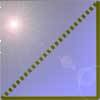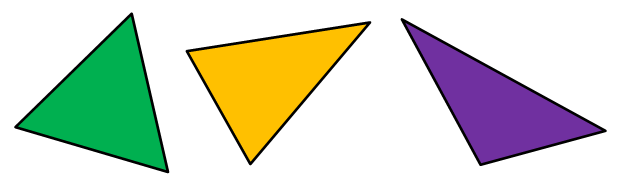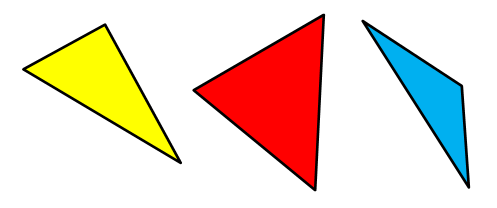#### You may also like### Tri.'s

How many triangles can you make on the 3 by 3 pegboard?### Cutting Corners

Can you make the most extraordinary, the most amazing, the most unusual patterns/designs from these triangles which are made in a special way?### Bracelets

Investigate the different shaped bracelets you could make from 18 different spherical beads. How do they compare if you use 24 beads?

# Name That Triangle!

##### Age 7 to 11Challenge Level

We normally name triangles by thinking about the lengths of the sides. If all the sides are equal, the triangle is an equilateral triangle. If two sides are the same length, we call the triangle an isosceles triangle. If none of the sides are the same length then this is a scalene triangle.

How would we name these triangles by thinking about the side lengths?You might also have heard about naming triangles using their angles. The name 'right-angled triangle' or just 'right triangle' is used for any triangle with one right angle. If a triangle has all acute angles, it is called an 'acute triangle'. If there are any obtuse angles in the triangle, this is an 'obtuse triangle'.

How would we name these triangles by thinking about the angle sizes?If you're stuck, you could put the triangles from this sheet into your table to start with, instead of drawing your own. Cut them out and look at one triangle at a time. Try rotating the triangle so that you can see it from different directions.

Look at the sides of the triangle - are any the same length as each other? How many are the same length? You might want to use a ruler to check!

Look at the angles of the triangle - are they all less than a right angle? Use the corner of a piece of paper to compare each angle to a right angle.